Interview Questions and Answers for 'Sopra Steria' | Search Java Interview Question - javasearch.buggybread.com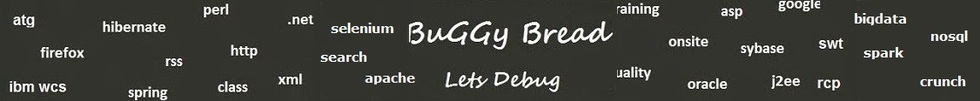# Search Interview Questions3361 questions in repository.There are more than 900 unanswered questions.Have a video suggestion.
Click Correct / Improve and please let us know.
Label / Company      Label / Company / TextSubmit Question

Interview Questions and Answers for 'Sopra Steria' - 3 question(s) found - Order By Newest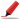Very frequently asked. Among first few questions in almost all interviews. Among Top 5 frequently asked questions. Frequently asked in Indian service companies (HCL,TCS,Infosys,Capgemini etc based on multiple feedback )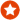Q1. Difference between == and .equals() ?Core Java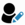Anonymous
Ans. "equals" is the method of object class which is supposed to be overridden to check object equality, whereas "==" operator evaluate to see if the object handlers on the left and right are pointing to the same object in memory.

x.equals(y) means the references x and y are holding objects that are equal. x==y means that the references x and y have same object.

Sample code:

String x = new String("str");
String y = new String("str");

System.out.println(x == y); // prints false
System.out.println(x.equals(y)); // prints true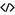Sample Code for equalsHelp us improve. Please let us know the company, where you were asked this question :LikeDiscussCorrect / Improvejava   string comparison   string   object class   ==    equals   object equality  operator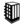Asked in 289 Companies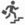basic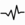frequent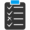Try 6 Question(s) TestRelated Questions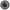What is the difference between = and == in Java ?How is == operator different for objects and primitive types ?What will be the output of following code

Integer x = 1;
Integer y = 2;
System.out.println(x == y);

What if you change 1 to "1" and Integer to String?Q2. Difference between Array and ArrayList ?Core Java2016-12-15 14:13:12Ans. <a href="http://javahungry.blogspot.com/2015/03/difference-between-array-and-arraylist-in-java-example.html" rel="nofollow">http://javahungry.blogspot.com/2015/03/difference-between-array-and-arraylist-in-java-example.html</a>Sample Code for ArrayListHelp us improve. Please let us know the company, where you were asked this question :LikeDiscussCorrect / Improvearray  arraylistAsked in 11 CompaniesbasicfrequentRelated QuestionsWhy Char array is preferred over String for storing password?If you are given a choice to use either ArrayList and LinkedList, Which one would you use and Why ?What is the difference between ArrayList and LinkedList ?Which of the following is false about main method ?

a. It should be declared public and static
b. it should have only 1 argument of type String array
c. We can override main method
d. We can overload main methodDifference between Vector and ArrayList ?What are the disadvantages of using arrays ?What are the Disadvantages of using Collection Classes over Arrays ?Will this code give error if i try to add two heterogeneous elements in the arraylist. ? and Why ?Difference between ArrayList and LinkedList ?Which of the following exception is thrown when we try to access element which is beyond the size ?Q3. Write a Program to print factorial of a number using recursionCore Java2017-01-29 17:47:20public static void main(String args[]) {
System.out.println(factorial(5));
}

private static int factorial(int number){
if(number == 1){
return 1;
} else {
return number * factorial(number - 1);
}
}
}Help us improve. Please let us know the company, where you were asked this question :LikeDiscussCorrect / ImproverecursionAsked in 24 CompaniesRelated QuestionsWhat will happen if we don't have termination statement in recursion ?Find Fibonacci first n numbers using recursion ?Write an Algorithm for Graph Traversal ? The Graph has a loop.Write a program to print sum of numbers between the start and end number

For example - Passing start number as 2 and end number as 10, it should print 2+3+4+5+6+7+8+9+10 = 54Write a program using Recursion to print multiplication of numbers between the start and end number

For example - Passing start number as 2 and end number as 10, it should print 2*3*4*5*6*7*8*9*10 = 3628800What is recursion in Java ?What will happen if we don't have termination statement in recursion ?Which of the two - iteration or recursion - is slower ?Write a Program to print fibonacci series using recursion.

## Help us and Others Improve. Please let us know the questions asked in any of your previous interview.

Any input from you will be highly appreciated and It will unlock the application for 10 more requests.